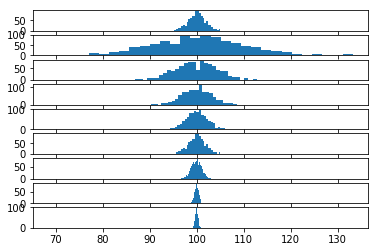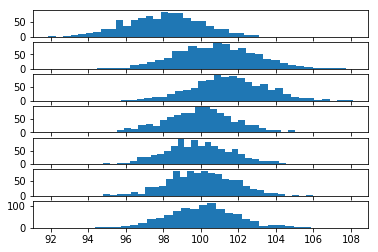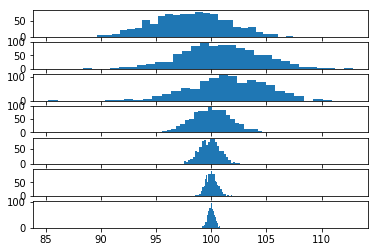In :
import math
import numpy as np
import pandas as pd
import matplotlib.pyplot as plt

In :
# We can infer the distribution of an expected value (ie. the confidence interval of the average observation)
# by sampling from the population of observations in multiple iterations. For each iteration we record the mean
# The result is a sample of means.
def bootstrap_mean(population, sample_size, num_samples):
sampled_expected_values = []
for i in range(num_samples):
sample = np.random.choice(a=population, size=sample_size, replace=True)
sampled_expected_values.append(np.average(sample))
return sampled_expected_values

In :
# Notice how the sample size affects our distribution of sampled means (ie bootstrapped confidence interval).
# Which sample size should be pick?
# Sample sizes of 1 and len(population) both give the correct expected value
# but fail to provide meaninful information about how confident we are about the expected value.
num_samples=1000
sample_sizes = [30, 1, 5, 10, 20, 30, 100, 500, population_size]
fig, axs = plt.subplots(len(sample_sizes), 1, sharex=True)

for i in range(0, len(sample_sizes)):
np.random.seed(15251)
population_size = 1000
population = np.random.normal(loc=100, scale=10, size=population_size)

sample_size = sample_sizes[i]
np.random.seed(65390)
sampled_mean = bootstrap_ci(population=population, sample_size=sample_size, num_samples=num_samples)
axs[i].hist(sampled_mean, bins=30)In :
# How does increasing the population size change the distribution of our sampled means
# (ie bootstrapped confidience intervals), assuming fixed sample size of 30?
# After a certain point it doesn't.
# Question to the reader: why doesn't doesn't the variance of the sampled means decrease with more evidence?
# Hint: Bootstrapping confidence intervals of expected values is distribution agnostic.
num_samples=1000
population_sizes= [10, 30, 100, 1000, 10000, 100000, 1000000 ]
fig, axs = plt.subplots(len(population_sizes), 1, sharex=True)

for i in range(0, len(population_sizes)):
np.random.seed(15251)
population_size = population_sizes[i]
population = np.random.normal(loc=100, scale=10, size=population_size)

np.random.seed(65390)
sampled_mean = bootstrap_ci(population=population, sample_size=30, num_samples=num_samples)
axs[i].hist(sampled_mean, bins=30)In :
# How can one overcome this issue of bootstrapped confidence intervals?
# I recommend making the sample size a function of the population size.
# This is an ugly engineering solution to an unsolvable problem.
# Setting sample_size=sqrt(population_size) creates the illusion that our confidence interval
# is shrinking.
num_samples=1000
population_sizes= [10, 30, 100, 1000, 10000, 100000, 1000000 ]
fig, axs = plt.subplots(len(population_sizes), 1, sharex=True)

for i in range(0, len(population_sizes)):
np.random.seed(15251)
population_size = population_sizes[i]
population = np.random.normal(loc=100, scale=10, size=population_size)

np.random.seed(65390)
sample_size = int(math.sqrt(population_size))
if sample_size < 10:
sample_size = 10
sampled_mean = bootstrap_ci(population=population, sample_size=sample_size, num_samples=num_samples)
axs[i].hist(sampled_mean, bins=30)In [ ]: